Back to Contents

# Putting Things in Boxes

So far, we have considered “pure” functions which have no side-effects, and functions which have the side-effect of reading or writing information to and from, for example, files. When we assigned a value to a name, that value could never change. Sometimes, it is convenient to allow the value of a name to be changed – some algorithms are more naturally expressed in this way.

OCaml provides a construct known as a reference which is a box in which we can store a value. We build a reference using the built-in function `ref` of type α α ref. For example, let us build a reference with initial contents 0. It will have type int ref.

` OCaml`

`# let x = ref 0;;`
`val x : int ref = {contents = 0}`

OCaml tells us that `x` is a reference of type int ref which currently has contents `0`. We can extract the current contents of a reference using the `!` operator, which has type α ref α.

`# let p = !x;;`
`val p : int = 0`

We can update the contents of the reference using the `:=`  operator:

`# x := 50;;`
`- : unit = ()`

The `:=`  operator has type α ref α unit, since it takes a reference and a new value to put in it, puts the value in, and returns nothing. It is only useful for its side-effect. Now, we can get the contents with `!` again.

`# let q = !x;;`
`val q : int = 50`
`# p;;`
`- : int = 0`

Notice that `p` is unchanged. Here’s a function to swap the contents of two references: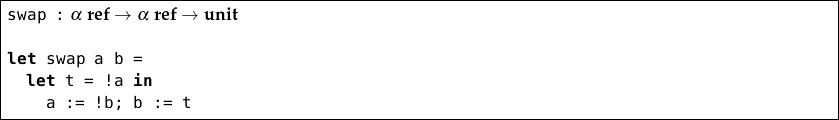We needed to use a temporary name `t` to store the contents of `a`. Can you see why?

This type of programming, which consists of issuing a number of commands, in order, about which references are to be altered and how, is known as imperative programming. OCaml provides some useful structures for imperative programming with references. We will look at these quickly now, and in a moment build a bigger example program to show why they are useful.

For readability, OCaml lets us miss out the `else` part of the `if` `…` `then` `…` `else` `…` construct if it would just be `()`, which is if we are doing nothing in the `else` case, so

`if`` x = 0 ``then`` a := 0 ``else`` ()`

can be written as

`if`` x = 0 ``then`` a := 0`

and if `x` is not zero, the expression will just evaluate to `()`. Due to this, when putting imperative code inside `if` `…` `then` `…` `else` `…` constructs, we need to surround the inner imperative expressions with parentheses so the meaning is unambiguous: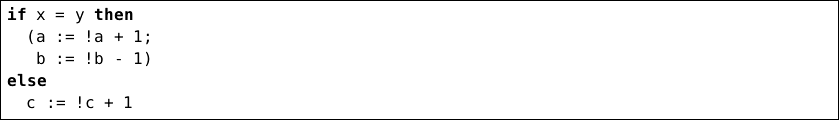OCaml allows us to use `begin` and `end` instead, for readability: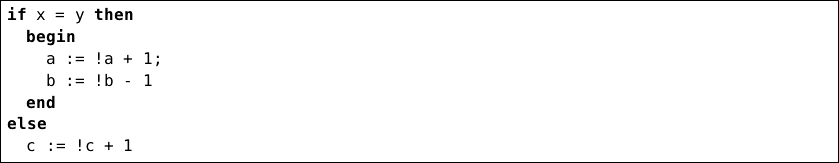## Doing it again and again

There are two ways to repeat an action. To perform an action a fixed number of times, we use the `for`` … = … ``to`` … ``do`` … ``done` construct. For example,

`for`` x = 1 ``to`` 5 ``do`` print_int x; print_newline () ``done`

evaluates the expression `print_int x; print_newline ()` five times: once where `x` is 1, once where `x` is 2 etc, so the result is:

`# for x = 1 to 5 do print_int x; print_newline () done;`
`1`
`2`
`3`
`4`
`5`
`- : unit = ()`

This is known as a “for loop”. Note that the type of the whole `for`` … = … ``to`` … ``do`` … ``done` expression is unit irrespective of the type of the expression(s) inside it.

There is another looping construct – this time for evaluating an expression repeatedly until some condition is true. This is the `while`` … ``do`` … ``done` construct. It takes a boolean condition, and evaluates a given expression repeatedly, zero or more times, until the boolean condition is `false`. For example, here is a function which, given a positive integer, calculates the lowest power of two greater than or equal to that number (i.e. for the argument 37, the result will be 64).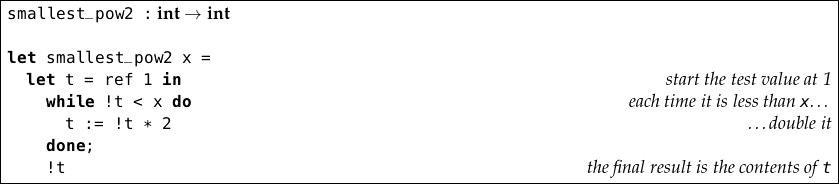The `while` loop continues until the contents of the reference `t` is greater than or equal to `x`. At that point, it ends, and the contents of `t` is returned from the function. Again, note that the type of the whole `while`` … ``do`` … ``done` construct is unit.

## Example: text file statistics

We are going to write a program to count the number of words, sentences and lines in a text file. We shall consider the opening paragraph of Kafka’s “Metamorphosis”.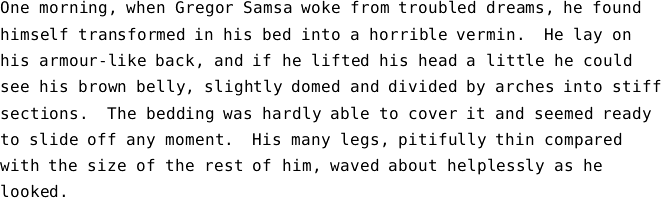There are newline characters at the end of each line, save for the last. You can cut and paste or type this into a text file to try these examples out. Here, it is saved as `gregor.txt`.

We will just count lines first. To this, we will write a function `channel_statistics` to gather the statistics by reading an input channel and printing them. Then we will have a function to open a named file, call our first function, and close it again.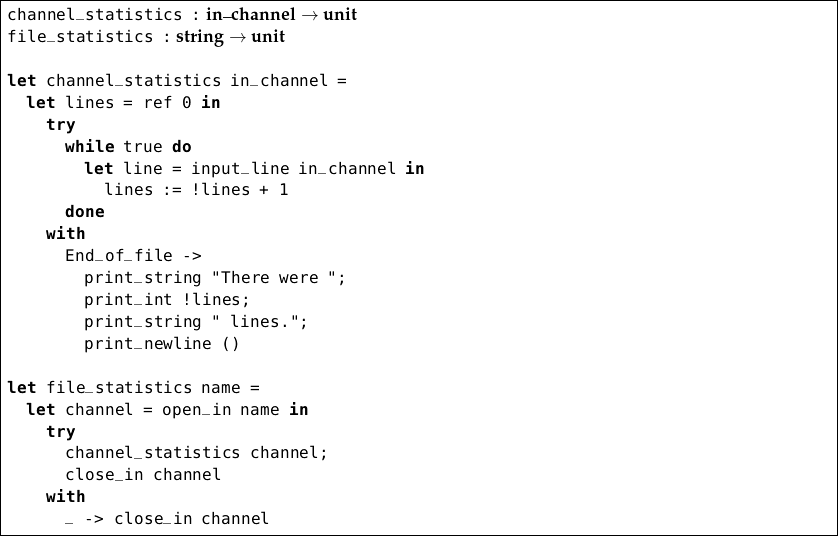Notice the use of `true` as the condition for the `while` construct. This means the computation would carry on forever, except that the `End_of_file` exception must eventually be raised. Note also that OCaml emits a warning when reading the `channel_statistics` function:

`Warning 26: unused variable line.`

This is an example of a warning we can ignore – we are not using the actual value `line` yet, since we are just counting lines without looking at their content. Running our program on the example file gives this:

` OCaml`

`# file_statistics "gregor.txt";;`
`There were 8 lines.`
`- : unit = ()`

Let us update the program to count the number of words, characters, and sentences. We will do this simplistically, assuming that the number of words can be counted by counting the number of spaces, and that the sentences can be counted by noting instances of `’.’`, `’!’`, and `’?’`. We can extend the `channel_statistics` function appropriately – `file_statistics` need not change: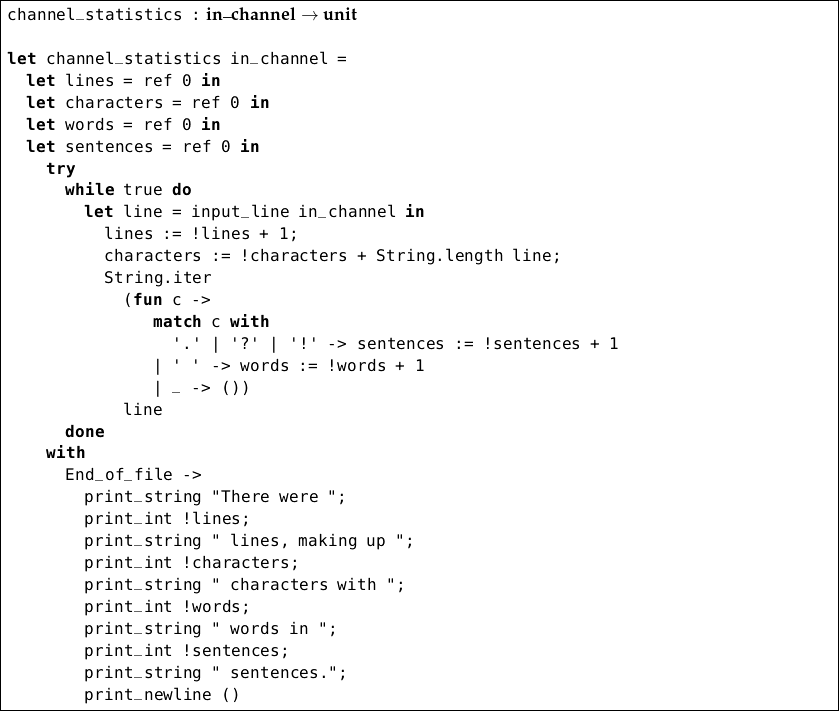We have used the built-in function `String.iter` of type (char unit) string unit which calls a function we supply on each character of a string.

Substituting this version of `channel_statistics` (if you are cutting and pasting into OCaml, be sure to also paste `file_statistics` in again afterwards, so it uses the new `channel_statistics`), gives the following result on our example text:

` OCaml`

`# file_statistics "gregor.txt";;`
`There were 8 lines, making up 464 characters with 80 words in 4 sentences.`
`- : unit = ()`

We should like to build a histogram, counting the number of times each letter of the alphabet or other character occurs. It would be tedious and unwieldy to hold a hundred or so references, and then pattern match on each possible character to increment the right one. OCaml provides a data type called array for situations like this.

An array is a place for storing a fixed number of elements of like type. We can introduce arrays by using `[|` and `|]`, with semicolons to separate the elements:

` OCaml`

`# let a = [|1; 2; 3; 4; 5|];;`
`val a : int array = [|1; 2; 3; 4; 5|]`

We can access an element inside our array in constant time by giving the position of the element (known as the subscript) in parentheses, after the array name and a period:

`# a.(0);;`
`- : int = 1`

Notice that the first element has subscript 0, not 1. We can update any of the values in the array, also in constant time, like this:

`# a.(4) <- 100;;`
`- : unit = ()`
`# a;;`
`a : int array = [|1; 2; 3; 4; 100|]`

If we try to access or update an element which is not within range, an exception is raised:

`# a.(5);;`
`Exception: Invalid_argument "index out of bounds".`

There are some useful built-in functions for dealing with arrays. The function `Array.length` of type α array int returns the length of an array:

`# Array.length a;;`
`- : int = 5`

In contrast to finding the length of a list, the time taken by `Array.length` is constant, since it was fixed when the array was created. The `Array.make` function is used for building an array of a given length, initialized with given values. It takes two arguments – the length, and the initial value to be given to every element. It has type int α α array.

`# Array.make 6 true;;`
`- : bool array = [|true; true; true; true; true; true|]`
`# Array.make 10 'A';;`
`- : char array = [|'A'; 'A'; 'A'; 'A'; 'A'; 'A'; 'A'; 'A'; 'A'; 'A'|]`
`# Array.make 3 (Array.make 3 5);;`
`- : int array array = [|[|5; 5; 5|]; [|5; 5; 5|]; [|5; 5; 5|]|]`

Back to our original problem. We want to store a count for each possible character. We cannot subscript our arrays with characters directly, but each character has a special integer code (its so-called “ASCII code”, a common encoding of characters as integers in use since the 1960s), and we can convert to and from these using the built-in functions `int_of_char` and `char_of_int`. For example:

` OCaml`

`# int_of_char 'C';;`
`- : int = 67`
`# char_of_int 67;;`
`- : char = 'C'`

The numbers go from 0 to 255 inclusive (they do not all represent printable characters, for example the newline character `’\n’` has code 10). So, we can store our histogram as an integer array of length 256.

Our main function is getting rather long, so we will write a separate one which, given the completed array prints out the frequencies. If there were no instances of a particular character, no line is printed for that character.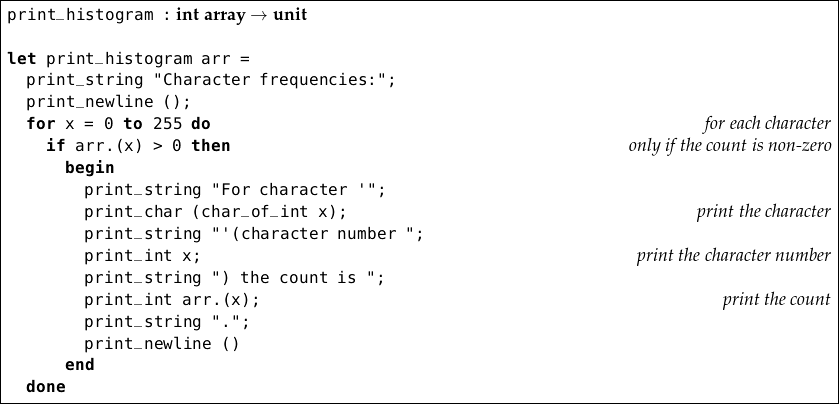This prints lines like:

`For character ’d’ (character number 100) the count is 6.`

Now, we can alter our `channel_statistics` to create an appropriate array, and update it, once again using `String.iter`: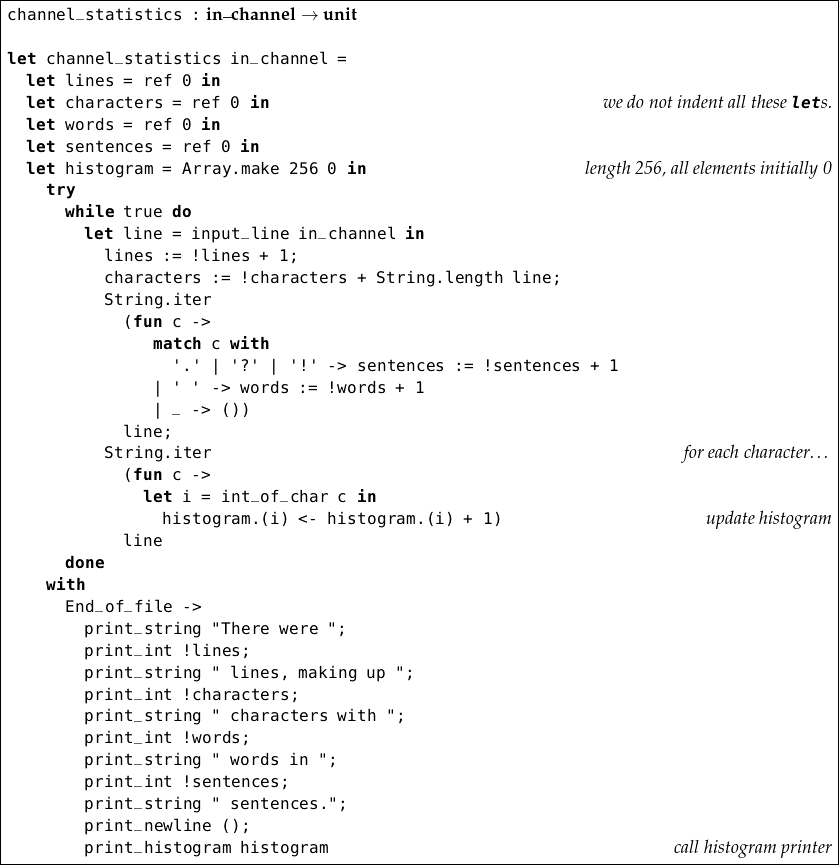Here is the output on our text:

` OCaml`

`# file_statistics "gregor.txt";;`
`There were 8 lines, making up 464 characters with 80 words in 4 sentences.`
`Character frequencies:`
`For character ' ' (character number 32) the count is 80.`
`For character ',' (character number 44) the count is 6.`
`For character '-' (character number 45) the count is 1.`
`For character '.' (character number 46) the count is 4.`
`For character 'G' (character number 71) the count is 1.`
`For character 'H' (character number 72) the count is 2.`
`For character 'O' (character number 79) the count is 1.`
`For character 'S' (character number 83) the count is 1.`
`For character 'T' (character number 84) the count is 1.`
`For character 'a' (character number 97) the count is 24.`
`For character 'b' (character number 98) the count is 10.`
`For character 'c' (character number 99) the count is 6.`
`For character 'd' (character number 100) the count is 25.`
`For character 'e' (character number 101) the count is 47.`
`For character 'f' (character number 102) the count is 13.`
`For character 'g' (character number 103) the count is 5.`
`For character 'h' (character number 104) the count is 22.`
`For character 'i' (character number 105) the count is 30.`
`For character 'k' (character number 107) the count is 4.`
`For character 'l' (character number 108) the count is 23.`
`For character 'm' (character number 109) the count is 15.`
`For character 'n' (character number 110) the count is 21.`
`For character 'o' (character number 111) the count is 27.`
`For character 'p' (character number 112) the count is 3.`
`For character 'r' (character number 114) the count is 20.`
`For character 's' (character number 115) the count is 24.`
`For character 't' (character number 116) the count is 21.`
`For character 'u' (character number 117) the count is 6.`
`For character 'v' (character number 118) the count is 4.`
`For character 'w' (character number 119) the count is 6.`
`For character 'y' (character number 121) the count is 10.`
`For character 'z' (character number 122) the count is 1.`
`- : unit = ()`

The most common character is the space. The most common alphabetic character is `’e’`.

## Questions

1. Consider the expression

`let`` x = ref 1 ``in` `let`` y = ref 2 ``in` `x := !x + !x; y := !x + !y; !x + !y`

What references have been created? What are their initial and final values after this expression has been evaluated? What is the type of this expression?

2. What is the difference between `[ref 5; ref 5]` and `let`` x = ref 5 ``in` `[x; x]`?

3. Imagine that the `for`` … = … ``to`` … ``do`` … ``done` construct did not exist. How might we create the same behaviour?

4. What are the types of these expressions?

`[|1; 2; 3|]`

`[|true; false; true|]`

`[|[|1|]|]`

`[|[1; 2; 3]; [4; 5; 6]|]`

`[|1; 2; 3|].(2)`

`[|1; 2; 3|].(2) <- 4`

5. Write a function to compute the sum of the elements in an integer array.

6. Write a function to reverse the elements of an array in place (i.e. do not create a new array).

7. Write a function `table` which, given an integer, builds the int array array representing the multiplication table up to that number. For example, `table 5` should yield: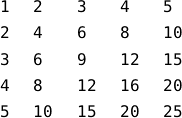There is more than one way to represent this as an array of arrays; you may choose.

8. The ASCII codes for the lower case letters `’a’``’z’` are 97…122, and for the upper case letters `’A’``’Z’` they are 65…90. Use the built-in functions `int_of_char` and `char_of_int` to write functions to uppercase and lowercase a character. Non-alphabetic characters should remain unaltered.

9. Comment on the accuracy of our character, word, line, and sentence statistics in the case of our example paragraph. What about in general?

10. Choose one of the problems you have identified, and modify our program to fix it.